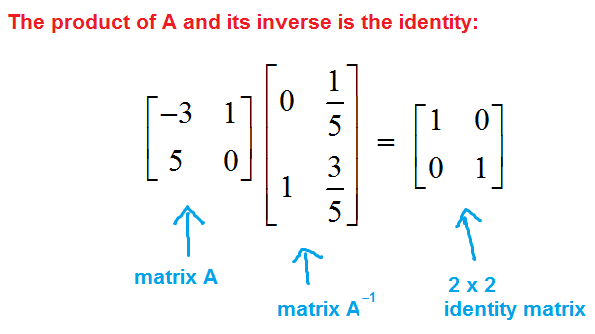# Invertible Matrix

## What is an Invertible Matrix?

An Invertible Matrix is a square matrix defined as invertible if the product of the matrix and its inverse is the identity matrix. An identity matrix is a matrix in which the main diagonal is all 1s and the rest of the values in the matrix are 0s. An invertible matrix is sometimes referred to as nonsingular or non-degenerate, and are commonly defined using real or complex numbers.Source

## How does an Invertible Matrix work?

The process of finding a matrix's inverse is known as matrix inversion. It is important to note, however, that not all matrices are invertible. For a matrix to be invertible, it must be able to be multiplied by its inverse. For example, there is no number that can be multiplied by 0 to get a value of 1, so the number 0 has no multiplicative inverse. Additionally, a matrix may have no multiplicative inverse, as is the case in matrices that are not square (different number of rows and columns).